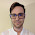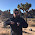Tuesday, May 12, 2015

Money defined as information mediation

In the previous post I wrote that "macroeconomics does not currently know what money is or does". Instead of simply tearing down, I'd like to be constructive. In the information equilibrium framework money has a rather simple and mathematically beautiful explanation.

Let's start with an AD/AS model with aggregate demand (N) in information equilibrium with aggregate supply (S) with the price level (P) as the detector. We write this model in information transfer notation as:

$$P : N \rightarrow S$$

and the information equilibrium condition

$$\text{(1) }\;\;\; P \equiv \frac{dN}{dS} = k \; \frac{N}{S}$$

In general we can make this transformation using a new variable M (i.e. money):

$$\text{(2) }\;\;\; P = \frac{dN}{dM} \frac{dM}{dS} = k \; \frac{N}{M} \frac{M}{S}$$

If we take N to be in information equilibrium with M, which is in information equilibrium with S, i.e.

$$P : N \rightarrow M \rightarrow S$$

Then we can use the information equilibrium condition

$$\frac{dM}{dS} = k_{s} \; \frac{M}{S}$$

to show that equation (2) can be re-written

$$P = \frac{dN}{dM} \frac{dM}{dS} = k \; \frac{N}{M} \frac{M}{S}$$

$$P = \frac{dN}{dM}\left( k_{s} \; \frac{M}{S} \right) = k \; \frac{N}{M} \frac{M}{S}$$

$$P = \frac{dN}{dM} = \frac{k}{k_{s}} \; \frac{N}{M}$$

$$\text{(3) }\;\;\; P = \frac{dN}{dM} = k_{n} \; \frac{N}{M}$$

Where we've defined $k_{n} \equiv k/k_{s}$. The solution to differential equation (3) defines a quantity theory of money where the price level goes as

$$\log P \sim ( k_{n} - 1 ) \log M$$

In words, we've introduced a new widget that mediates the information transfer from aggregate demand to aggregate supply, which allows us to re-write the entire theory in terms of aggregate demand and the quantity of that widget (i.e. money).

One interesting side note -- if we consider non-ideal information transfer we have to combine the equations:

$$\text{(4a) }\;\;\; \frac{dM}{dS} \leq k_{s} \; \frac{M}{S}$$

$$\text{(4b) }\;\;\; P = \frac{dN}{dM} \frac{dM}{dS} \leq k \; \frac{N}{M} \frac{M}{S}$$

Since by equation (4a) the derivative $dM/dS$ is less than $k_{s} M/S$, so the replacement of the derivative is with a quantity that is greater than the derivative. Therefore, we don't have the bound and in general we have to allow

$$\frac{dN}{dM} \nleq k_{n} \; \frac{N}{M}$$

That is to say, out of information equilibrium, the price level can be above or below the equilibrium value given by the quantity theory of money (as is observed) -- with disequilibrium on the supply side being key to above equilibrium inflation. Supply shocks (supply out of equilibrium with money) tend to lead to inflation (e.g. the oil shocks of the 1970s) while demand shocks (demand out of equilibrium with money) tend to lead to disinflation.

Update 5/13/2015:

A couple more observations:

• It is interesting that the information transfer index $k_{n}$, which becomes $1/\kappa$ in the price level model I use, is a composite of the indices $k/k_{s}$. The index $k$, representing a 'conversion factor' from aggregate supply to aggregate demand and the index $k_{s}$, representing the conversion factor from aggregate supply to money, should in a sense be of the same order -- meaning the index $k_{n}$ should be approximately ~ 1. Which is what we observe ($k_{n}$ seems to range from about 0.9 to 2.5, or $\kappa$ from 0.4 to 1.1).
• A liquidity trap economy has $k_{s} \approx k$, i.e. $\kappa \approx 1$, while a quantity theory economy has $k > k_{s}$, i.e. $\kappa < 1$.

1.I've always been confused about how you have the price level proportional to to N/S when the definition of real GDP is nominal GDP divided by the price level. That should mean that P = N/S...

1.Hi John,

There is an overall scale freedom in defining P (e.g. the year where P = 100).

But also S here is not exactly RGDP -- it is widgets of aggregate supply and has units of items (10 cars, 16 toasters, 6 massages ... 10 pounds of stuff).

For a cooler reason (from effective field theory in physics), see here. In short, if I am defining a theory that obeys long run neutrality such that $N \rightarrow \alpha N$ and $S \rightarrow \alpha S$ stays the same, the simplest equation I can write with dynamics is:

$$\frac{dN}{dS} = k \; \frac{N}{S}$$

which stays the same under that transformation. Any $k$ is allowed there so it must be allowed in the theory.

There is probably something even cooler involving anomalous scaling that maybe I should look into ...